# 图神经网络的表示方法和使用案例

2021/08/26 09:16

## GNN 的正式表示方法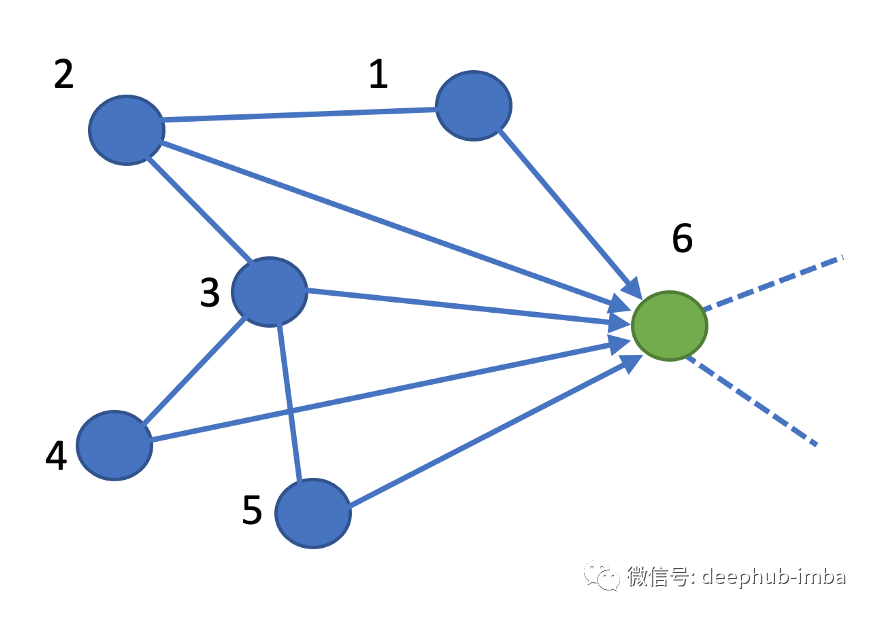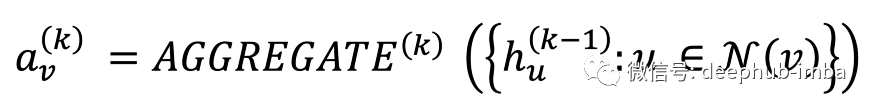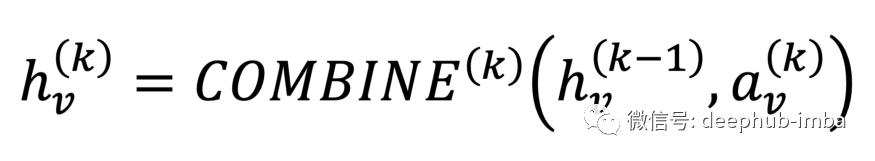## PyTorch几何框架

GNN 可以使用 pytorch 几何库轻松实现。你可以找到许多 GNN 的实现和一个消息传递类来使用你自己的自定义实现。在以下链接中查看。

Cora 数据集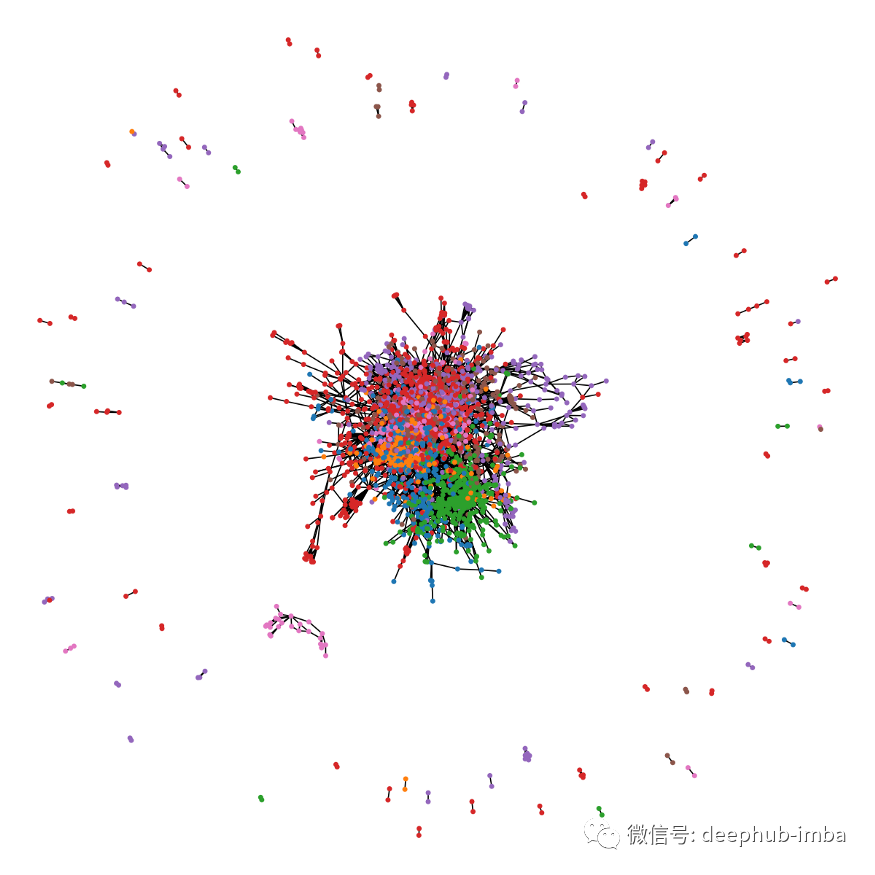UMAP查看特征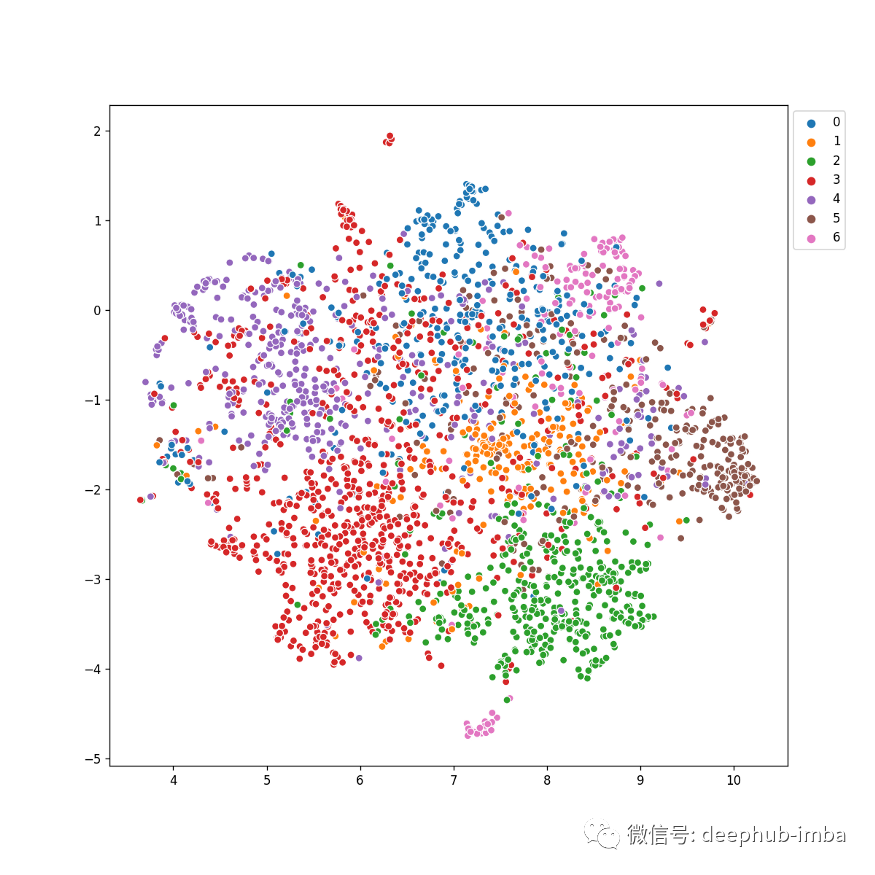# essential imports that will be needed throughout the blog import torch import torch.nn.functional as F from torch_geometric.datasets import Planetoid from torch_geometric.nn import GCNConv import matplotlib.pyplot as plt import seaborn as sns import umap import networkx as nx import numpy as npdataset = 'Cora' path = "./" dataset = Planetoid(path, dataset, transform=T.NormalizeFeatures()) data = datasetembd = umap.UMAP().fit_transform(data.x.numpy()) plt.figure(figsize=(10, 10)) sns.scatterplot(x=embd.T, y=embd.T, hue=data.y.numpy(), palette=palette) plt.legend(bbox_to_anchor=(1,1), loc='upper left')

 class Net(torch.nn.Module):     def __init__(self):         super(Net, self).__init__()         self.conv1 = GCNConv(dataset.num_features, 16, cached=True)         self.conv2 = GCNConv(16, 16, cached=True)                  self.fc1 = torch.nn.Linear(16, dataset.num_classes)         def forward(self):         x, edge_index, edge_weight = data.x, data.edge_index,                                           data.edge_attr         x = self.conv1(x, edge_index, edge_weight)         x = F.relu(x)         x = F.dropout(x, training=self.training)         x = self.conv2(x, edge_index, edge_weight)         x = F.relu(x)         x = F.dropout(x, training=self.training)         x = self.fc1(x)                  return F.log_softmax(x, dim=1)   

 device = torch.device('cuda' if torch.cuda.is_available() else 'cpu') model, data = Net().to(device), data.to(device) optimizer = torch.optim.Adam([     dict(params=model.conv1.parameters(), weight_decay=5e-4),     dict(params=model.fc1.parameters(), weight_decay=5e-4),     dict(params=model.conv2.parameters(), weight_decay=0) ], lr=0.01) def train():     model.train()     optimizer.zero_grad()     F.nll_loss(model()[data.train_mask],                    data.y[data.train_mask]).backward()     optimizer.step()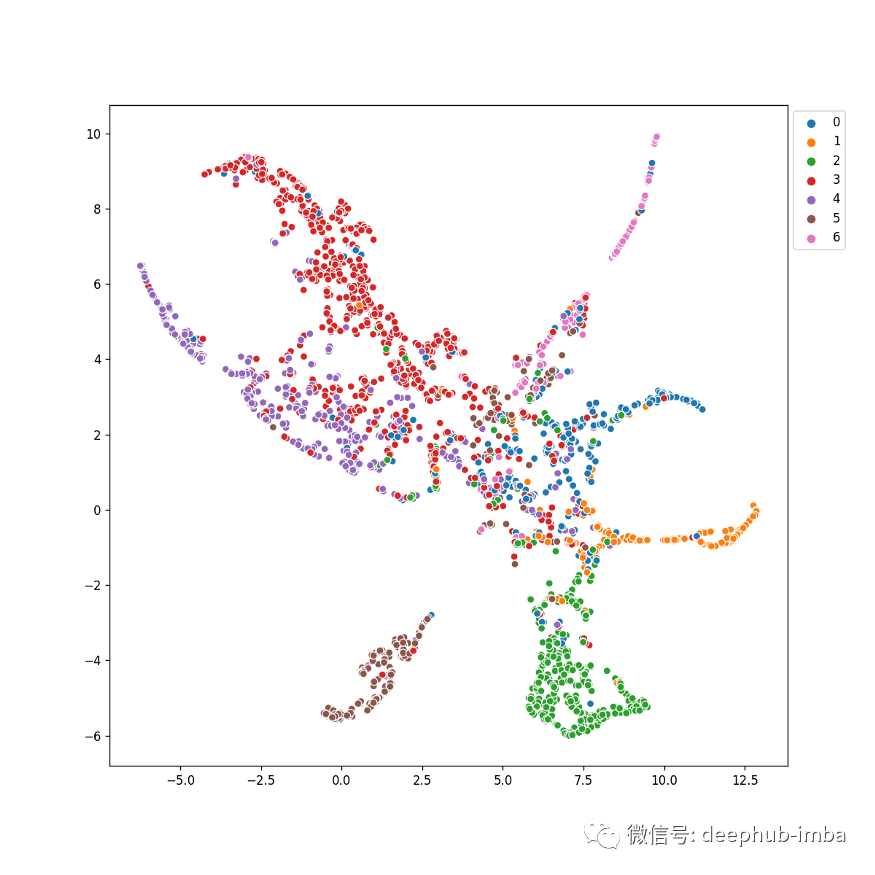## Embedding losses

1. 要求 GNN 更紧密地嵌入相似的类

2. 要求 GNN 进一步嵌入不同的类

 y_neg_pairs = [] y_pos_pairs = []  data_idx = np.arange(len(data.x)) for idx1, y1 in enumerate(data.y[data.train_mask].cpu().numpy()):     for idx2, y2 in enumerate(data.y[data.train_mask].cpu().numpy()):         if idx1 > idx2 and y1!=y2:             y_neg_pairs.append([idx1, idx2])         if idx1 > idx2 and y1==y2:             y_pos_pairs.append([idx1, idx2])              y_neg_pairs = np.array(y_neg_pairs) y_pos_pairs = np.array(y_pos_pairs)

 model_out = model()[data.train_mask]     y_true = data.y[data.train_mask]     nllloss = F.nll_loss(model_out, y_true)          #Negative loss     disloss_neg = F.logsigmoid(-1 * (model_out[y_neg_pairs.T]*model_out[y_neg_pairs.T])).sum(-1).mean()          #Positive loss     disloss_pos = F.logsigmoid((model_out[y_pos_pairs.T]*model_out[y_pos_pairs.T])).sum(-1).mean()          loss = 10 * nllloss - disloss_neg - disloss_pos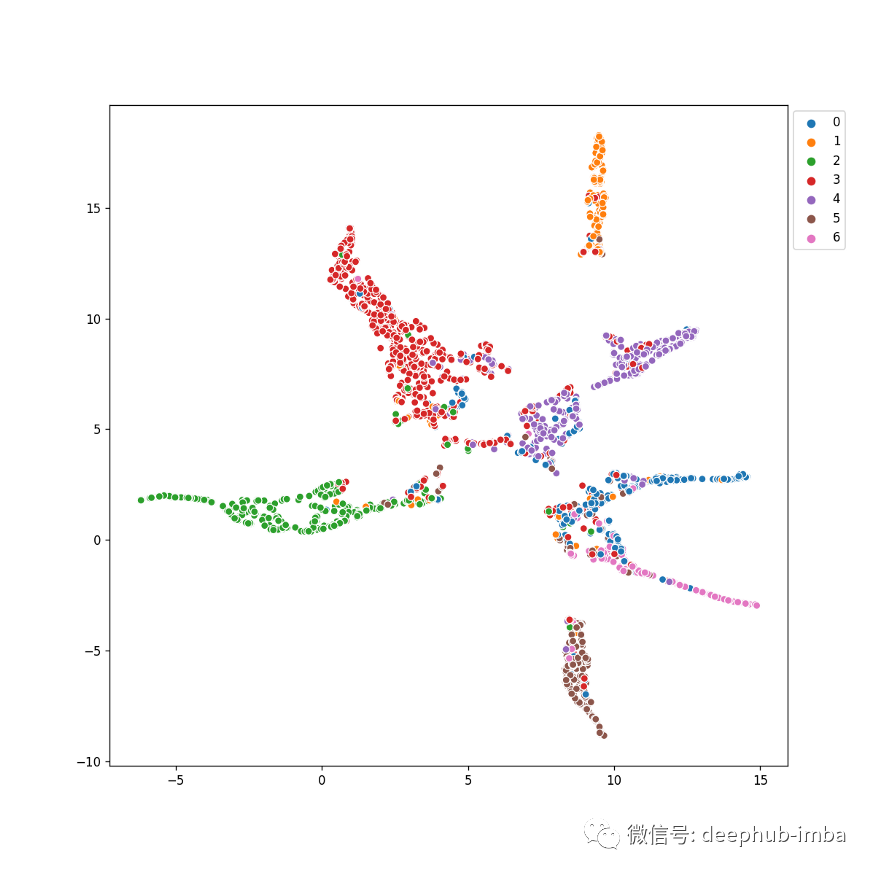## 将想法扩展到无监督聚类0 评论
0 收藏
0